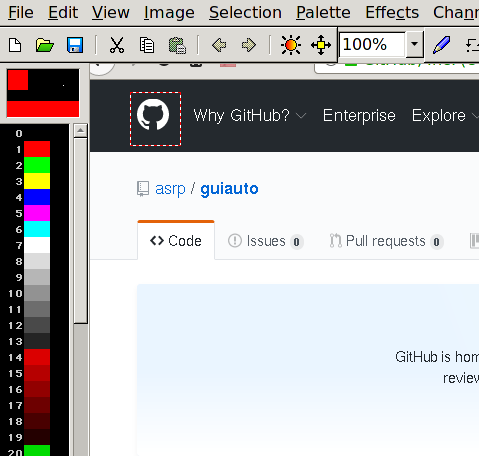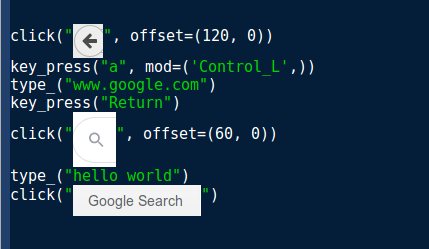# Roll your own GUI automation library

Sikuli is a tool for automating repetitive tasks.To automate a program, it makes use of screenshots and image recognition to decide where to click and type [automation_methods]. As you can see above, Sikuli uses Python for its script's language (plus rendered image). But under the hood, its implemented in Java and runs Jython. Since nothing Sikuli uses really needs Java, we'll try to implement some GUI automation in pure Python.

## Overview

We want to write a library so that calling something like

``````click("path/to/image.png")
``````

will find a copy of `image.png` on the screen and click it.

There are three components to this kind of GUI automation:

• taking screenshots
• image recognition
• input generation

This post assumes we're running on Linux, but similar tools for other platforms and even OS agnostic libraries do exist.

## Taking screenshots

We'll just use an existing program to take screenshots as a user. In this case mtpaint because its lightweight, but any screenshot program will do.

``````mtpaint -s
``````Then we can select a rectangle, hit the Delete key and save.

Our program also needs to take screenshots of the entire screen to find `image.png` on it. We'll do this with the Xlib library.

``````from Xlib import display, X

def screencap(x, y, w, h):
root = display.Display().screen().root
raw = root.get_image(x, y, w, h, X.ZPixmap, 0xffffffff)
return numpy.fromstring(raw.data, dtype=numpy.uint8).reshape(h, w, 4)[:, :, :3]
``````

we can get the current screen's width and height with

``````screen = display.Display().screen()
width, height = screen.width_in_pixels, screen.height_in_pixels
``````

## Image recognition

Sikuli uses a computer vision library, OpenCV, to find `image.png` within a screenshot. We'll do the same

``````import cv2

def find_best(needle, haystack):
match = cv2.matchTemplate(haystack, needle, cv2.TM_CCOEFF_NORMED)
min_val, max_val, min_loc, max_loc = cv2.minMaxLoc(match)
return max_loc, max_val
``````

Here, `needle` is `image.png` represented as a width by height by 3 (colours). `numpy` array. `haystack` is the screenshot represented in the same way.

`find_best` returns a position and a confidence value (between 0 and 1).

## Generating input events

To move the mouse and click, we'll use Xlib's xtest extension.

``````from Xlib import display, X
from Xlib.ext.xtest import fake_input

def mouse_move(x, y):
disp = display.Display()
root = disp.screen().root
root.warp_pointer(x, y)
disp.sync()

def mouse_click(button=1):
disp = display.Display()
fake_input(disp, X.ButtonPress, button)
disp.sync()
fake_input(disp, X.ButtonRelease, button)
disp.sync()
``````

## Combining everything

We now have all ingredients needed and are ready to implement `click("path/to/image.png")`.

``````import numpy

def find_on_screen(image):
screen = display.Display().screen()
width, height = screen.width_in_pixels, screen.height_in_pixels
haystack = numpy.array(screencap(0, 0, width, height))
return needle.shape[1::-1], find_best(needle, haystack)

def click(image, similarity=0.7):
shape, (pos, confidence) = find_on_screen(image)
if confidence > similarity:
center = [int(p + s/2) for p, s in zip(pos, shape)]
mouse_move(*center)
mouse_click()
``````

We used `cv2.imread` to load the image from its path and if OpenCV finds a copy of the image on screen with high enough confidence (here 70% confidence by default) then click on the middle of the image.

The combined source code is here.

## Improvements

We've got a usable starting point that we can improve a bit. Or you could stop reading here and make your own changes.

The first thing we might notice is that full screen captures takes a while to complete.

### Faster screenshots

We can use a C function that returns an array of size `width` times `height` times 3 (colours) from pixels on the screen. We'll first make it a flat array and reshape it later [c_screenshot].

``````//Compile hint: gcc -shared -O3 -lX11 -fPIC -Wl,-soname,prtscn -o prtscn.so prt
#include <X11/X.h>
#include <X11/Xlib.h>

void getScreen(const int, const int, const int, const int, unsigned char *);
void getScreen(const int xx, const int yy, const int w, const int h, unsigned char * output)
{
Display *display = XOpenDisplay(NULL);
Window root = DefaultRootWindow(display);

XImage *image = XGetImage(display,root, xx, yy, w, h, AllPlanes, ZPixmap);

int x, y;
int ii = 0;
for (y = 0; y < h; y++) {
for (x = 0; x < w; x++) {
unsigned long pixel = XGetPixel(image, x, y);
output[ii++] = (pixel & image->red_mask) >> 16;
output[ii++] = (pixel & image->green_mask) >> 8;
}
}
XDestroyImage(image);
XDestroyWindow(display, root);
XCloseDisplay(display);
}
``````

Compiling this will give us a `screencap.so` shared library file that we can call from Python.

``````import ctypes
import numpy

lib_filename = '/abspath/to/screencap.so'
c_screencap = ctypes.CDLL(lib_filename)
c_screencap.getScreen.argtypes = []

def screencap(x, y, w, h):
objsize = w * h * 3

# result = c_screencap.getScreen(x, y, w, h)
result = (ctypes.c_ubyte * objsize)()
c_screencap.getScreen(x, y, w, h, result)

return numpy.array(result, numpy.uint8).reshape([h, w, 3])
``````

### Keyboard input

We can use xtest to generate keyboard events. However, we have to pass a keycode which is inconvenient. Given a `display.Display`, we can lookup the keycode from a key's name (as a string) like so:

``````from Xlib import XK

def string_to_keycode(d, key):
return d.keysym_to_keycode(XK.string_to_keysym(key))
``````

and we can press keys with the result

``````def press_key(key, mod=()):
d = display.Display()
keycode = string_to_keycode(d, key)
fake_input(d, X.KeyPress, keycode)
d.sync()
fake_input(d, X.KeyRelease, keycode)
d.sync()
``````

We can wrap this inside a function for typing an entire string.

``````char_to_key = defaultdict({" ": "space", ".": "period"})

def type_(s):
for key in s:
press_key(char_to_key.get(key, key))
``````

### Seeing images in the editor

In Sikuli, we see images instead of filenames in our editor which makes it immediately clear where we are trying to move our mouse pointer to. But our implementation only shows filenames.I happen to use Emacs which has an iimage minor mode. Enabling it with `M-x iimage-mode` gives the resultsNote that this doesn't automatically reload images when the path to a new file is typed.

Unfortunately, this solution is Emacs specific and any solution here would be tied to a specific editor (either existing or new).